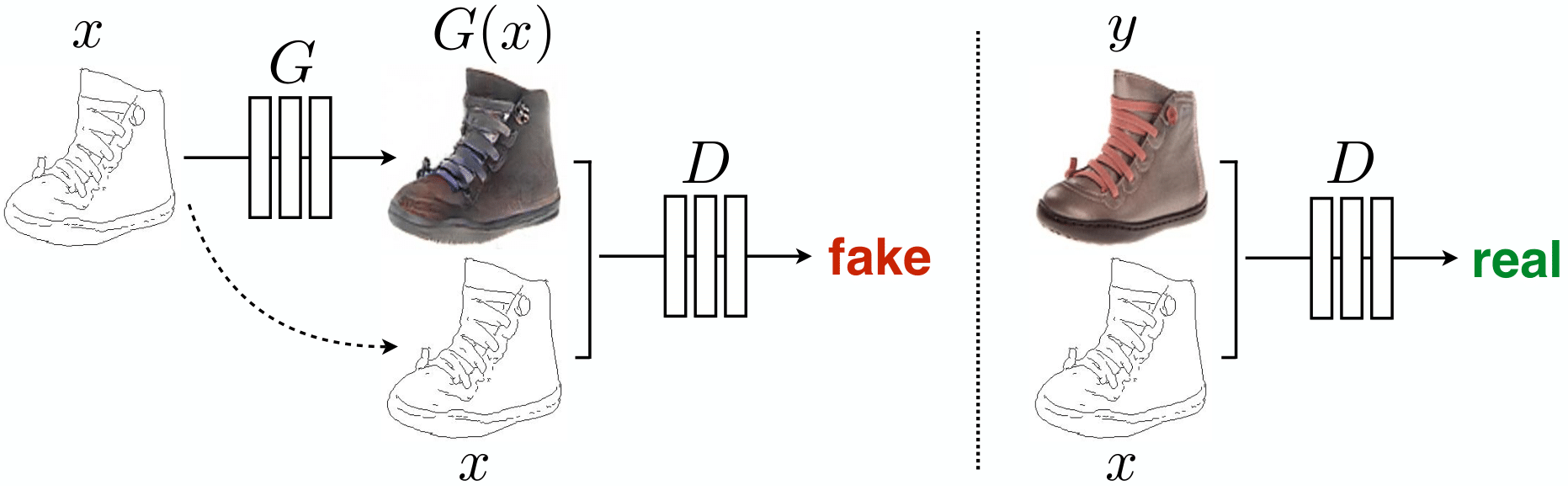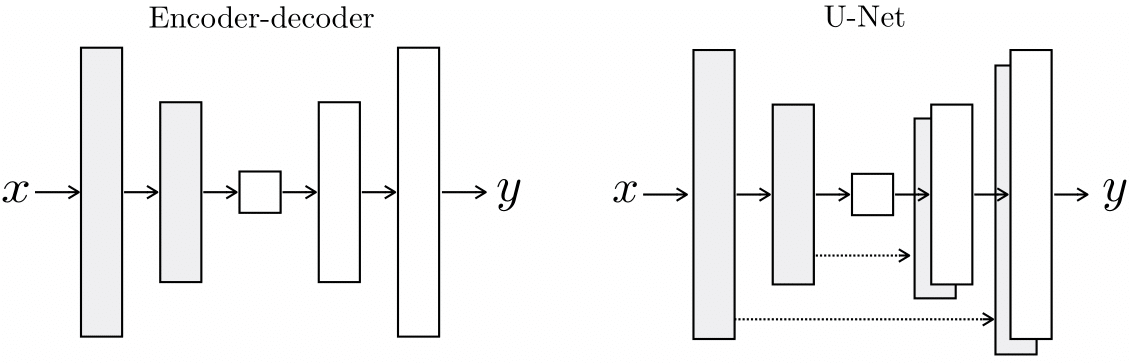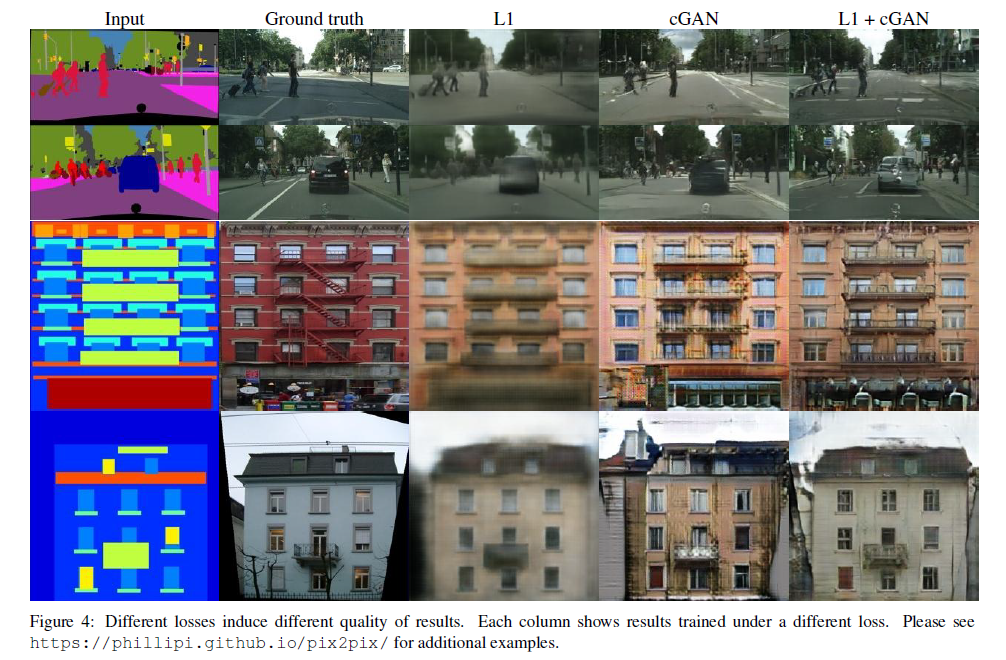# Image-to-Image Translation with Conditional Adversarial Networks• First general purpose conditional GAN for image to image translation task
• Impressive output on inpainting, future state prediction, image manipulation guided by user constraints, style transfer, super-resolution

# Method

• U-net based architecture
• PatchGAN classifier

Generator $G$ of conditional GAN is denoted as $G: \{x,z\} \rightarrow y$ when $x$ is conditional input and $z$ is random vector

## objective

conditional GAN objective can be denoted as followings:

$\mathcal{L}_{cGAN} (G,D) = \mathbb{E}_{x,y}[\log D(x,y)] + \mathbb{E}_{x,z}[\log (1-D(x,G(x,z))]$

In this way, Generator and Discriminator both get conditional input.

As previous approaches show that mixing GAN objective with traditional loss, authors of this paper also used L1 distance too.

$\mathcal{L}_{L1}(G) = \mathbb{E}_{x,y,z}[{\lVert {y-G(x,z)} \rVert} _1]$

So, final objective is derived as followings:

$G^* = \arg\min_G\max_D \mathcal{L}_{cGAN}(G,D) + \lambda \mathcal{L}_{L1}(G).$

### noise $z$

Without $z$, the generator could learn deterministic outputs because the generator is only conditioned by conditional input $x$.

But the authors say that they found adding Gaussian noise $z$ to $x$ is not effective, as the network just ignores noise $z$.

So they used dropout to some layers to provide noise. Despite the dropout noise, There is only minor stochasticity in the output of the network. In this paper, the way that makes conditional GANs produce highly stochastic output is not provided

## Network Architectures

Both generator and discriminator use modules of the form convolution-BatchNorm-ReLU• Generator: U-net shape Encoder / Decoder and skip connection

### Markovian discriminator (PatchGAN)In image generation task, Generator with L2 loss or L1 loss can produce blurry outputs. In other words, L2, L1 loss make model concentrate on low frequency area and this concentration can fail on high frequency crispness. So, model with L1/L2 loss, produces blurry Image.

This paper used both L1 loss and GAN discriminator loss. They entrust correcting at low frequencies to L1 loss and correcting at high frequencies to discriminator loss. For high frequency area, it is sufficient to pay attention to the local image patch. So the authors made “PatchGAN” in which discriminator only classify whether small image patch looks like real or not. Model penalize high frequency area by using PatchGAN convolutionally and averaging all PatchGAN’s penalties. It is demonstrated that the size of the patch is much smaller than a full image.